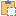#### BoundarySegment Class

An object that represents a segment of an area boundary.

Namespace: Autodesk.Revit.DB
Assembly: RevitAPI (in RevitAPI.dll) Version: 16.0.0.0 (16.0.0.0)

# Syntax

C#
``public class BoundarySegment : IDisposable``
Visual Basic
``````Public Class BoundarySegment _
Implements IDisposable``````
Visual C++
``public ref class BoundarySegment : IDisposable``

# Remarks

These objects define the exterior boundary of an area. The geometry of the segment can be retrieved along with the id of the element that is responsible for producing that boundary.

# ExamplesCopy C#
``````public void GetInfo_BoundarySegment(Room room)
{
IList<IList<Autodesk.Revit.DB.BoundarySegment>> segments = room.GetBoundarySegments(new SpatialElementBoundaryOptions());

if (null != segments)  //the room may not be bound
{
string message = "BoundarySegment";
foreach (IList<Autodesk.Revit.DB.BoundarySegment> segmentList in segments)
{
foreach (Autodesk.Revit.DB.BoundarySegment boundarySegment in segmentList)
{

// Get curve start point
message += "\nCurve start point: (" + boundarySegment.GetCurve().GetEndPoint(0).X + ","
+ boundarySegment.GetCurve().GetEndPoint(0).Y + "," +
boundarySegment.GetCurve().GetEndPoint(0).Z + ")";
// Get curve end point
message += ";\nCurve end point: (" + boundarySegment.GetCurve().GetEndPoint(1).X + ","
+ boundarySegment.GetCurve().GetEndPoint(1).Y + "," +
boundarySegment.GetCurve().GetEndPoint(1).Z + ")";
// Get document path name
message += ";\nDocument path name: " + room.Document.PathName;
// Get boundary segment element name
if (boundarySegment.ElementId != ElementId.InvalidElementId)
{
message += ";\nElement name: " + room.Document.GetElement(boundarySegment.ElementId).Name;
}
}
}
}
}``````Copy VB.NET
``````Public Sub GetInfo_BoundarySegment(room As Room)
Dim segments As IList(Of IList(Of Autodesk.Revit.DB.BoundarySegment)) = room.GetBoundarySegments(New SpatialElementBoundaryOptions())

If segments IsNot Nothing Then
'the room may not be bound
Dim message As String = "BoundarySegment"
For Each segmentList As IList(Of Autodesk.Revit.DB.BoundarySegment) In segments
For Each boundarySegment As Autodesk.Revit.DB.BoundarySegment In segmentList

' Get curve start point
message += ((vbLf & "Curve start point: (" + boundarySegment.GetCurve().GetEndPoint(0).X & ",") + boundarySegment.GetCurve().GetEndPoint(0).Y & ",") + boundarySegment.GetCurve().GetEndPoint(0).Z & ")"
' Get curve end point
message += ((";" & vbLf & "Curve end point: (" + boundarySegment.GetCurve().GetEndPoint(1).X & ",") + boundarySegment.GetCurve().GetEndPoint(1).Y & ",") + boundarySegment.GetCurve().GetEndPoint(1).Z & ")"
' Get document path name
message += ";" & vbLf & "Document path name: " + room.Document.PathName
' Get boundary segment element name
If boundarySegment.ElementId IsNot ElementId.InvalidElementId Then
message += ";" & vbLf & "Element name: " + room.Document.GetElement(boundarySegment.ElementId).Name
End If

Next
Next